# NCERT Solutions for Class 7 Maths Chapter 9 Rational Numbers| PDF Download

On this page, you will find Chapter 9 Rational Numbers Class 7 Maths NCERT Solutions which will help you in attaining good marks in the examinations and complete homework on time. You can download PDF of Chapter 9 Rational Numbers NCERT Solutions which will give good experience and provide opportunities to learn new things. Chapter 9 NCERT Solutions will serve as beneficial tool that can be used to recall various questions any time.

Class 7 Maths NCERT Solutions will be useful in understanding the concepts of the chapter properly. These NCERT Solutions are helpful resources that can help you not only cover the entire syllabus but also inculcate correct learning habits among students.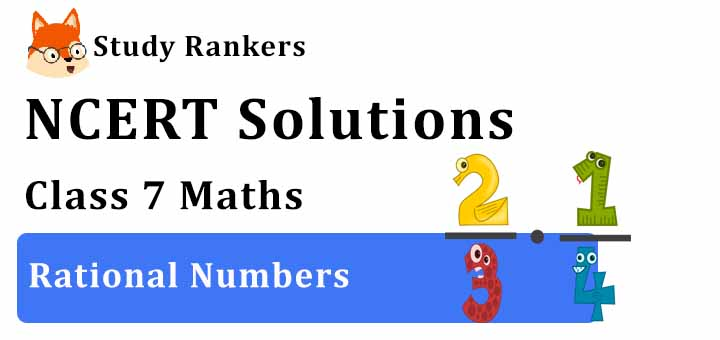Exercise 9.1

1. List five rational numbers between:
(i) -1 and 0
(ii) -2 and - 1
(iii) -4/5 and -2/3
(iv) -1/2 and 2/3

(i) -1 and 0
Let us write -1 and 0 as rational numbers with denominator 6.Therefore, five rational numbers between -1 and 0 would be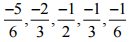(ii) -2 and -1
Let us write -2 and -1 as rational numbers with denominator 6.Therefore, Five rational numbers between -2 and -1 would be(iii) -4/5 and -2/3
Let us write -4/5 and -2/3 as rational numbers with the same denominators.Therefore, five rational numbers between -4/5 and -2/3 would be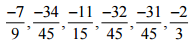(iv) -1/2 and 2/3
Let us write -1/2 and 2/3 as rational numbers with the same denominators.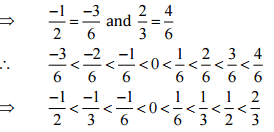Therefore, five rational numbers between -1/2 and 2/3 would be2. Write four more rational numbers in each of the following patterns: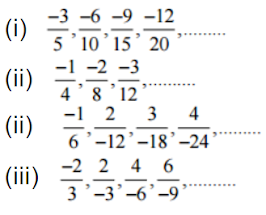Therefore, four equivalent rational numbers are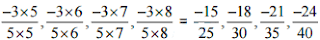(ii)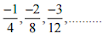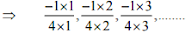Therefore, the next four rational numbers of this pattern would be(iii)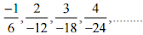Therefore, the next four rational numbers of this pattern would be(iv)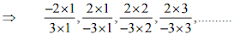Therefore, the next four rational numbers of tins pattern would be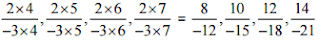3. Give four rational numbers equivalent to:
(i) -2/7
(ii) 5/-3
(iii) 4/9
(iv) -2/7

(i)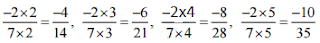Therefore, four equivalent rational numbers are(ii) 5/-3Therefore, four equivalent rational numbers are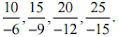(iii) 4/9Therefore, four equivalent rational numbers are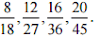4. Draw the number line and represent the following rational numbers on it:
(i) 3/4
(ii) -5/8
(iii) -7/4
(iv) 7/8

(i) 3/4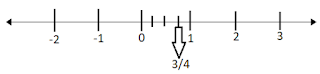(ii) -5/8(iii)  -7/4(iv) 7/8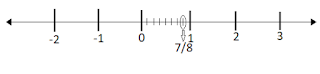5. The points P, Q, R, S, T, U, A and B on the number line are such that, TR = RS = SU and AP = PQ = QB. Name the rational numbers represented by P, Q, R and S.Therefore,SimilarlyThus, the rational numbers represented P, Q, R and S arerespectively.

6. Which of the following pairs represent the same rational numbers:(i)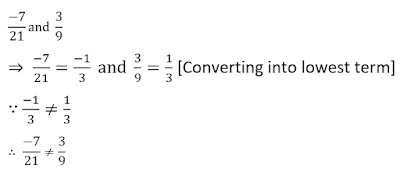(ii)(iii)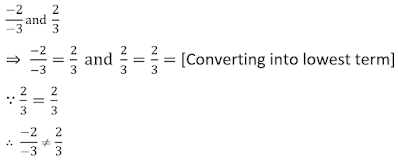(iv)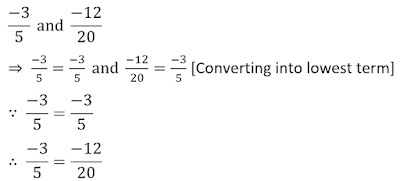(v)(vi)(vii)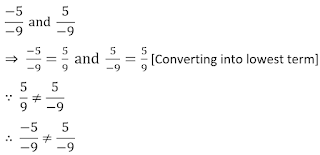7. Rewrite the following rational numbers in the simplest form:
(i) -8/6
(ii) 25/45
(iii) -44/72
(iv) -8/108. Fill in the boxes with the correct symbol out of <, > and =: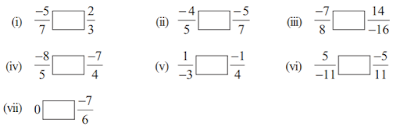9. Which is greater in each of the following: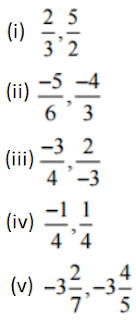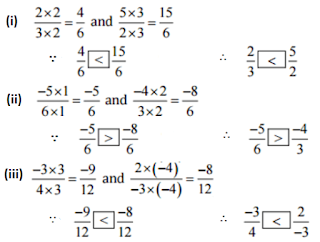10. Write the following rational numbers in ascending order:Exercise 9.2

1. Find the sum: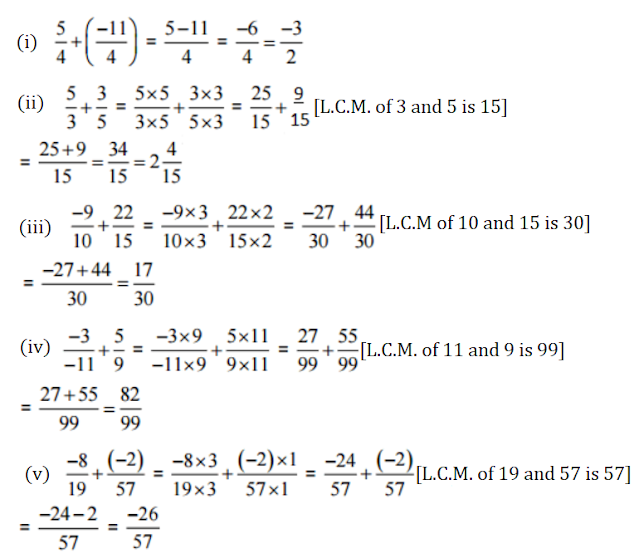2. Find: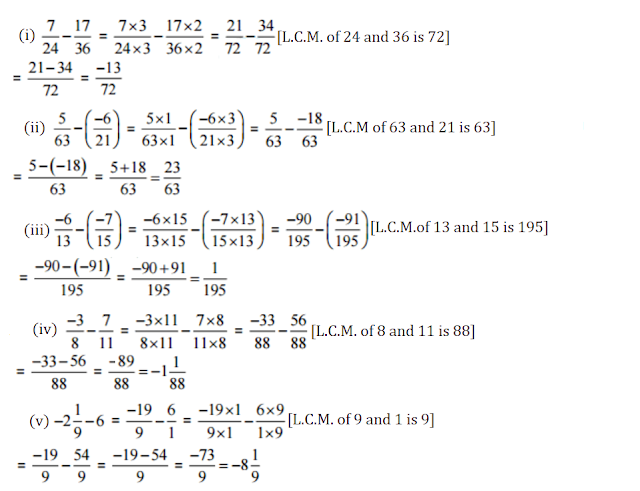3. Find the product: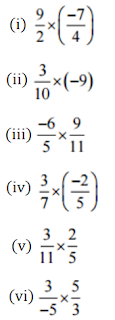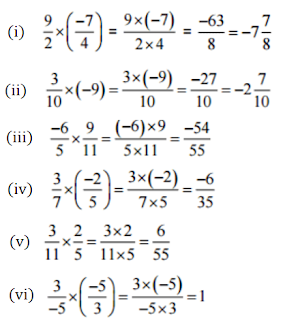4. Find the value of:## NCERT Solutions for Class 7 Maths Chapter 9 Rational Numbers

In Chapter 7 Maths NCERT Solutions, we will deal with rational numbers which is defined as a number that can be expressed in the form p/q , where p and q are integers and q ≠ 0. All numbers, including whole numbers, integers, fractions and decimal numbers, can be written in the numerator-denominator form.

• The rational number zero is neither negative nor positive. Positive rational numbers are represented to the right of 0. Negative rational numbers are represented to the left of 0.

• A rational number is said to be in its standard form if its numerator and denominator have no common factor other than 1, and its denominator is a positive integer.

You can get exercisewise NCERT Solutions just by clicking on the links given below which will help you in improving experience. It can be used to enrich knowledge and make lessons for learners more exciting.

You will get NCERT Solutions which are prepared by Studyrankers experts which will set a good foundation for your future goals. It is very challenging to socre good marks in tests that is why we have prepared NCERT Solutions.

### NCERT Solutions for Class 7 Maths Chapters:

 Chapter 1 Integers Chapter 2 Fractions and Decimals Chapter 3 Data Handling Chapter 4 Simple Equations Chapter 5 Lines and Angles Chapter 6 The Triangle and its Properties Chapter 7 Congruence of Triangles Chapter 8 Comparing Quantities Chapter 10 Practical Geometry Chapter 11 Perimeter and Area Chapter 12 Algebraic Expressions Chapter 13 Exponents and Powers Chapter 14 Symmetry Chapter 15 Visualising Solid Shapes

FAQ on Chapter 9 Rational Numbers

#### How many exercises are there in Chapter 9 Rational Numbers Class 7 Maths NCERT Solutions?

Chapter 9 Rational Numbers consists of only two exercises which will help you in improving the marks in the examinations and have edge over your classmates. It will help you in identify, analyze, and then rectify the mistakes.

#### Arrange -7/8, -5/6, -3/4 in the ascending order.

-7/8 < -5/6 < -3/4.

#### How to do addition of rational numbers?

• If the denominators of the given rational numbers are the same, then the denominator of their sum will also be the same. The numerator of the sum of two rational numbers with the same denominator is the sum of the numerators of the given numbers.
• To add rational numbers with different denominators, we convert them into equivalent rational numbers with the same denominator.
• Two rational numbers whose sum is zero are called additive inverses of each other.

#### How to do multiplication of rational numbers?

• To multiply two rational numbers, we simply multiply their numerators and denominators with their correct signs.
• Two rational numbers whose product is 1 are called reciprocals of each other.
• A rational number and its reciprocal will always have the same sign.